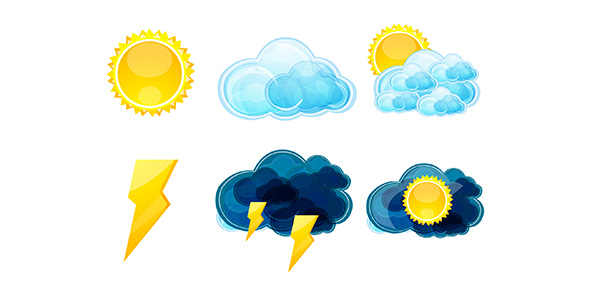# Taylor's Weather Quiz

33 QuestionsSettingsRelated Topics
• 1.
Warm Front: A warm air mass slides ___a____ and ___b____ a cold air mass to form a boundary. This usually brings __c____ and ___d___ weather.
• 2.
Cold Front: Cold air      a      under      b      air to form a boundary. This creates      c      and      d      weather and often thunderstorms.
• 3.
Air masses form when air remains over a region long enough to acquire the ___a____ and ___b__ of that region. Air masses move __c___ but do not __d__.
• 4.
Air pressure is determined by several factors including the ____a_____ of the air and warm and cold ___b____.
• 5.
Fronts are a ___a__ between 2 air masses of different __b___.
• 6.
• A.

Layer

• B.

Heap

• C.

Curl

• D.

Heavy

• 7.
Which of the following is not a type of cloud?
• A.

Cumulus

• B.

Stratus

• C.

Cirrus

• D.

All of the above are clouds

• 8.
Which type of cloud is fluffy, puffy, & big?
• A.

Cumulus

• B.

Nimbus

• C.

Cirrus

• D.

Stratus

• 9.
Which clouds signal fair weather?
• A.

The thin wispy cloud

• B.

The flat and layered cloud

• C.

The gray dark cloud

• D.

Puffy and big cloud

• 10.
• A.

Stratus

• B.

Cumulus

• C.

Cirrus

• D.

Nimbus

• 11.
Stratus clouds lay where/how in the sky?
• A.

High/fluffy

• B.

Low/flat like a blanket

• C.

High/wispy

• D.

Low/fluffy

• 12.
Which clouds cause light rain/snow?
• A.

Stratus

• B.

Cirrus

• C.

Nimbus

• D.

Cumulonimbus

• 13.
• A.

Stratus

• B.

Cumulus

• C.

Cirrus

• D.

Nimbus

• 14.
Cirrus means what?
• A.

Fluffy

• B.

Layered

• C.

High

• D.

Curl

• 15.
Cirrus clouds are. . .
• A.

Dark

• B.

Thin & Wispy

• C.

Lay like a blanket

• D.

Fluffy

• 16.
This cloud is seen in clear skies and good weather. .
• A.

Cirrus

• B.

Stratus

• C.

Cumulus

• D.

Nimbus

• 17.
• A.

Change in the weather

• B.

Snow

• C.

Rain

• D.

Fair Weather

• 18.
Which cloud means rain cloud?
• A.

Cumulus

• B.

Cirrus

• C.

Nimbus

• D.

Stratus

• 19.
Which clouds bring HEAVY rain?
• A.

Nimbus

• B.

Cirrus

• C.

Stratus

• D.

Cumulus

• 20.
• A.

Nimbo/stratus = storm/wispy

• B.

Nimbo/stratus = storm/fluffy

• C.

Nimbo/stratus = storm/layer

• D.

Nimbo/stratus = rain/curl

• 21.
• A.

Cotton Candy

• B.

Ice Crystals

• C.

Rain

• D.

Snow

• 22.
Air pressure is due to the ______ of the air.
• 23.
• A.

Wind

• B.

Weight of the air

• C.

Clouds

• D.

Barometer

• 24.
Which of the following determines air pressure?
• A.

Temperature of the air

• B.

Warm/Cold Fronts

• C.

They both determine air pressure

• 25.
A meterologist uses a ______ to measure air pressure.
• 26.
• A.

Thermometer

• B.

Anemometer

• C.

Barometer

• D.

Rain Gauge

• 27.
• A.

Evaporation

• B.

Condensation

• C.

Precipitaion

• 28.
• A.

Measures the temperature of air

• B.

Measures how much rain has fallen

• C.

Measures the speed of wind

• D.

Measures the weight of air

• 29.
• A.

Measure the speed of wind

• B.

Measure the amount of rain fallen

• C.

Measure the weight of air

• D.

Measure the temperature

• 30.
• A.

Measure the speed of wind

• B.

Measure the weight of air

• C.

Measure the temperature

• D.

Measure the amount of rain fallen

• 31.
• A.

Measure the speed of wind

• B.

Measure the amount of rain fallen

• C.

Measure the temperature

• D.

Measure how much the air weighs